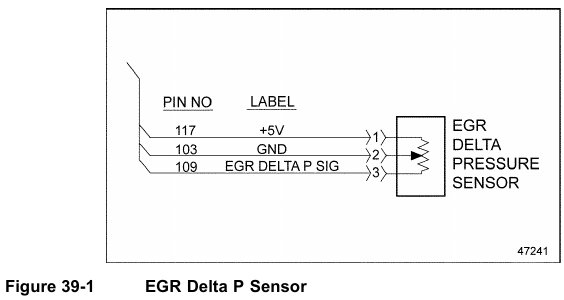# Series 60 – SPN 411/FMI 3 – EGR DIFFERENTIAL PRESSURE OR DELTA P SENSOR CIRCUIT OUTSIDE OF NORMAL OPERATING RANGE

This diagnosis is typically EGR Delta P Sensor circuit failed high.
39.2.1
Open Circuit Check
Check as follows:
1. Disconnect the EGR Delta P Sensor.
2. Measure the resistance across pins 1 and 3 of the EGR Delta P Sensor.
[a] If the resistance is greater than 130 kΩ, replace the EGR Delta P Sensor.
Refer to section 39.2.1.1.
[b] If the resistance is less than 130 kΩ, go to step 3.
3. Measure the resistance between pins 1 and 2 of the EGR Delta P Sensor.
[a] If the resistance is greater than 130 kΩ, replace the EGR Delta P Sensor.
Refer to section 39.2.1.1.
[b] If the resistance is less than 130 kΩ,, go to step 4.
4. Turn the ignition ON (key ON, engine OFF).
5. Measure the voltage between pins 1 and 2 of the EGR Delta P Sensor harness connector.
[a] If the voltage is between 4.5 and 5.5 volts, go to step 7.
[b] If the voltage is less than 4.5 volts, go to step 6.
6. Measure the voltage between pin 1 of the EGR Delta P Sensor harness connector and
ground.
[a]
If the voltage is between 4.5 and 5.5 volts, repair the open circuit between pin 2 of the
EGR Delta P Sensor harness connector and pin 103 of the 120–pin MCM connector.
See Figure 39-1. Refer to section 39.2.1.1.[b] If the voltage is less than 4.5 volts, repair the open circuit between pin 1 of the EGR
Delta P Sensor harness connector and pin 117 of the 120–pin MCM connector.
Refer to section 39.2.1.1.
7. Turn the ignition OFF.
8. Disconnect the 120–pin MCM connector.
9. Measure the resistance between pins 1 and 3 of the EGR Delta P Sensor harness connector.
[a] If the resistance is less than 5 Ω, repair the short in wires between pins 1 and 3 of
the EGR Delta P Sensor harness connector and pins 117 and 109 of the 120–pin
MCM connector. Refer to section 39.3.1.1.
[b] If the resistance is greater than 5 Ω, go to step 10.
10. Measure the resistance across pins 2 and 3 of the EGR Delta P Sensor harness connector.
[a] If the resistance is less than 5 Ω, repair the short in wires between pins 109 and 103
of the 120–pin MCM connector. Refer to section 39.3.1.1
[b] If the resistance is greater than 5 Ω, go to step 11.
11. Measure the resistance between pin 1of the EGR Delta P Sensor harness connector and ground.
[a] If the resistance is less than 5 Ω, repair the short circuit between pin 117 of the
120–pin MCM connector and ground. Refer to section 39.3.1.1.
[b] If the resistance is greater than 5 Ω, go to step 12.
12. Measure the resistance between pin 3 of the EGR Delta P Sensor harness connector and
ground.
[a] If the resistance is less than 5 Ω, repair the short between pin 109 of the 120–pin
MCM connector and ground. Refer to section 39.2.1.1.
[b] If the resistance is greater than 5 Ω, repair the open circuit between pin 3 of the
EGR Delta P Sensor harness connector and pin 109 of the 120–pin MCM connector.
Refer to section 39.2.1.1.

39.2.1.1
Verify Repairs
Verify repairs as follows:
1. Turn ignition OFF.
2. Reconnect any electrical connections that were disconnected to perform the diagnosis.